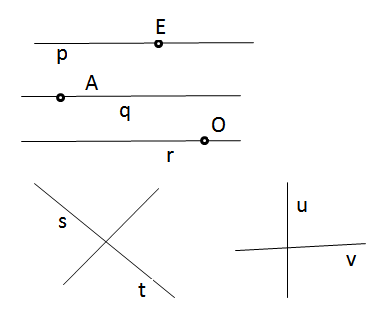# Can two line segments intersect each other at two points?

Solution:

Intersecting lines

Two lines in a plane, if they are not parallel, intersect in a point.

Two lines or line segments cannot intersect each other in two points. They do so only in one point;

In the figure below p, q, and r are parallel lines and E, A, and O are points on these parallel lines respectively.

There are two pairs of intersecting lines s and t; u and v.

Both of them intersect in a point.Updated on: 10-Oct-2022

85 Views